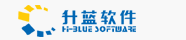# JScript 方法 - lbound 方法

March 25,2004

safeArray.lbound(dimension)

safeArray

dimension

<SCRIPT LANGUAGE="VBScript">
<!--
Function CreateVBArray()
Dim i, j, k
Dim a(2, 2)
k = 1
For i = 0 To 2
For j = 0 To 2
a(j, i) = k
k = k + 1
Next
Next
CreateVBArray = a
End Function
-->
</SCRIPT>

<SCRIPT LANGUAGE="JScript">
<!--

function VBArrayTest(vba)

{

var i, s;
var a = new VBArray(vba);
for (i = 1; i <= a.dimensions(); i++)
{
s = "The lower bound of dimension ";
s += i + " is ";
s += a.lbound(i)+ ".<BR>";
return(s);
}
}

-->

</SCRIPT>

<BODY>

<SCRIPT language="jscript">

document.write(VBArrayTest(CreateVBArray()));
</SCRIPT>

</BODY>